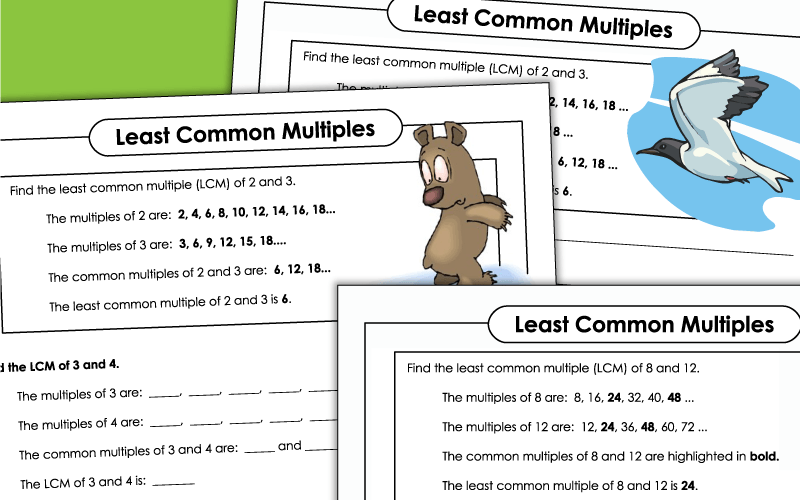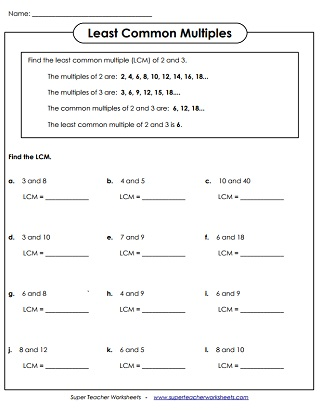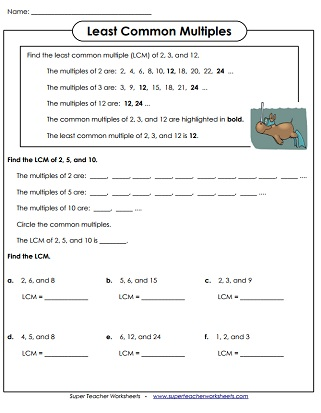# Least Common Multiple - LCM

Use these worksheets when teaching students to calculate the least common multiple, or LCM, of a group of numbers.## LCM of 2 Numbers

Another worksheet in which students determine the least common multiple for  each number pair.
Step-by-step practice sheet for finding the LCM of 2 numbers.
Students find the LCM for each number set, then answer the short-answer thinking questions.
This file contains 15 least common multiple activity cards. Use them for learning centers, peer review, exit slips, classroom scavenger hunts, or on your Smartboard's document camera.

## LCM of 3 Numbers

Factors and GCF

Factor tree worksheets and greatest common factor

Multiples

On these printable worksheets, students will identify multiples of the given numbers.

Simplifying fractions, equivalent fractions, and comparing fractions

Multiplication Worksheets

Games and worksheets that teach basic multiplication facts

## Sample Worksheet ImagesMy Account
Site Information